# Graph Data Structure - Variants or Types or Kinds

### <<Previous Next >>

In this page, we will learn about various types or kinds of graph.

### Graph Variants or Types of Graph

Directed Graph (or) Digraph: It is graph in which each edge has a direction to its successor.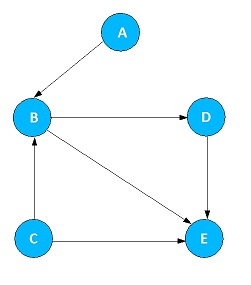Fig 1. Directed Graph

Undirected Graph: It is a graph in which there is no direction on the edges. The flow between two vertices can go in either direction.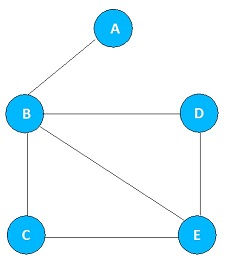Figure 2. Undirected Graph

Weighted Graph: If the graph has a some cost or weight on the edge, then we say that graph is said to be a weighted graph. Weight can be applied in both Directed and Undirected graph.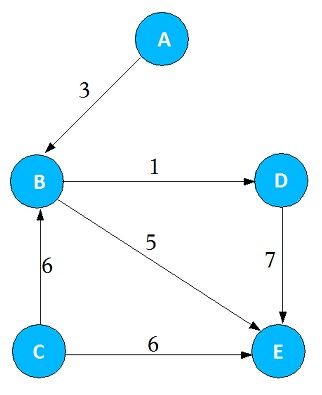Figure 3. Weighted Directed Graph

Unweighted Graph: If there is no cost or weight on the edge, then we say that graph is an Unweighted Graph. For example, Figure 2 is the Unweighted Undirected graph

### Based on How Connected the Graph is

Strongly Connected Graph: If there is a path from each vertex to every other vertex in the directed graph, then only we say that directed graph is said to be Strongly connected graph.

Weakly Connected Graph: If there are at least two vertices that are not connected, then we say that directed graph is said to be weakly connected graph.

Disjoint Graph: The graph is a disjoint, if it is not connected.

Complete Graph: If there is an edge between every pair of vertices, then we say that graph is said to be complete graph.

### Acyclic Graph

A graph is acyclic if it has no cycles.

Directed Acyclic Graph: A directed acyclic graph is directed graph without any directed cycles. Referred by its short name DAG

### PolyTree

PolyTree is a directed graph without any undirected cycles

### Forest

Forest is a undirected graph without any cycles

# Graph Data Structure - Variants or Types or Kinds

### <<Previous Next >>

In this page, we will learn about various types or kinds of graph.

### Graph Variants or Types of Graph

Directed Graph (or) Digraph: It is graph in which each edge has a direction to its successor.Fig 1. Directed Graph

Undirected Graph: It is a graph in which there is no direction on the edges. The flow between two vertices can go in either direction.Figure 2. Undirected Graph

Weighted Graph: If the graph has a some cost or weight on the edge, then we say that graph is said to be a weighted graph. Weight can be applied in both Directed and Undirected graph.Figure 3. Weighted Directed Graph

Unweighted Graph: If there is no cost or weight on the edge, then we say that graph is an Unweighted Graph. For example, Figure 2 is the Unweighted Undirected graph

### Based on How Connected the Graph is

Strongly Connected Graph: If there is a path from each vertex to every other vertex in the directed graph, then only we say that directed graph is said to be Strongly connected graph.

Weakly Connected Graph: If there are at least two vertices that are not connected, then we say that directed graph is said to be weakly connected graph.

Disjoint Graph: The graph is a disjoint, if it is not connected.

Complete Graph: If there is an edge between every pair of vertices, then we say that graph is said to be complete graph.

### Acyclic Graph

A graph is acyclic if it has no cycles.

Directed Acyclic Graph: A directed acyclic graph is directed graph without any directed cycles. Referred by its short name DAG

### PolyTree

PolyTree is a directed graph without any undirected cycles

### Forest

Forest is a undirected graph without any cycles

### << Previous Next >>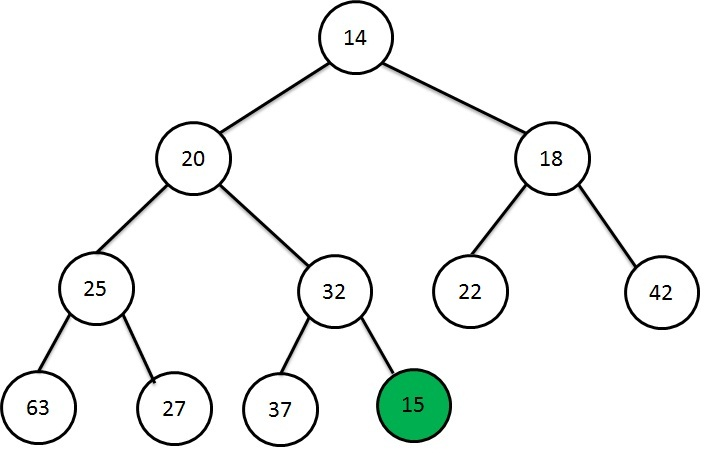Heap Data Structure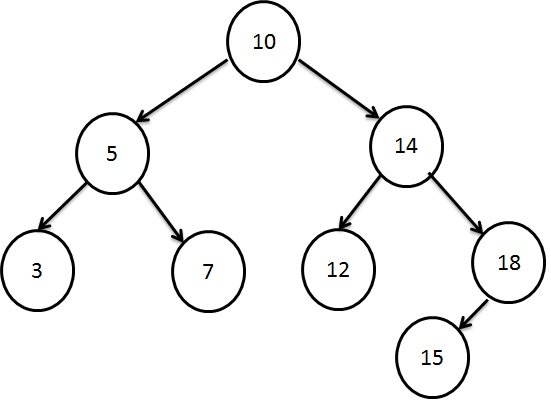Searching using Binary Search Tree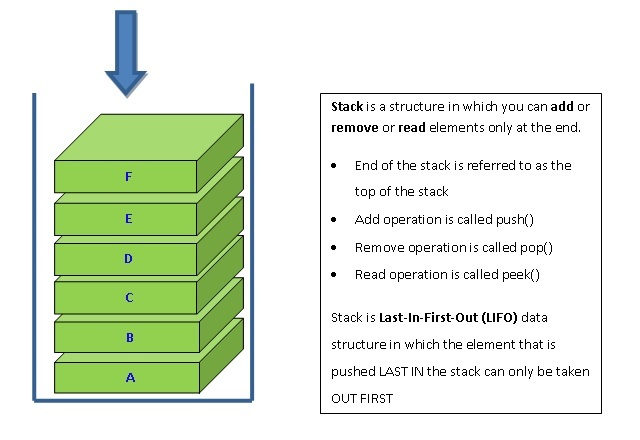Stacking the Data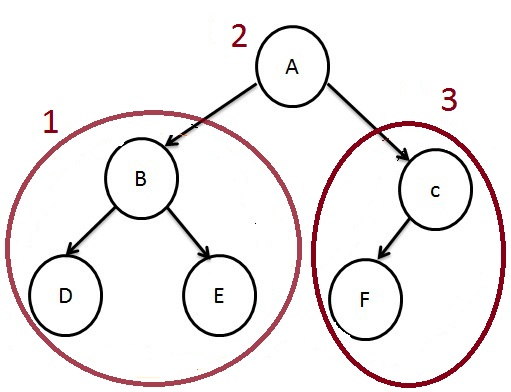How to traverse a Tree?